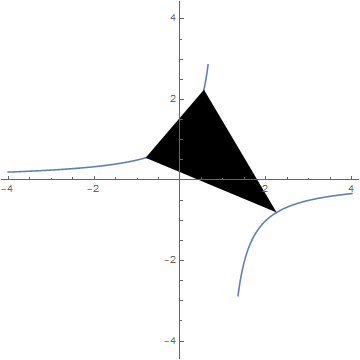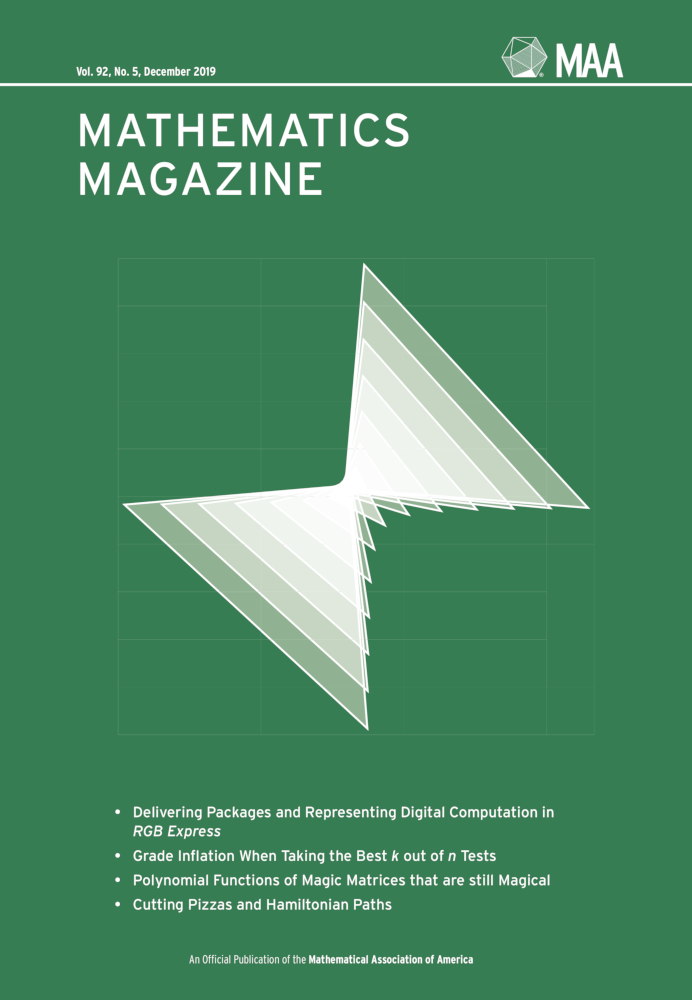Ramanujan's Butterfly Digital Print 2019

A family of thirteen triangles with vertices based on the roots of the Ramanujan simple cubic. All of the vertices of these triangles lie along the graph of the function $$n(x) = \frac{1}{1-x}$$.

### Background and Inspiration

Let $$r_1$$, $$r_2$$, and $$r_3$$ be the roots of the Ramanujan simple cubic $f_B(x) = x^3 - \left(\frac{3+B}{2}\right) x^2 - \left(\frac{3-B}{2}\right) x + 1,$ then the function $$n(x) = \frac{1}{1-x}$$ permutes the roots: $n(r_1) = r_2, \quad n(r_2) = r_3, \quad\text{and}\quad n(r_3) = r_1.$ I realized that because the function $$n(x)$$ permuted the roots, the three possible pairings $$(r_i,n(r_i))$$ would create triangles. Furthermore, the triangle vertices lie along the graph of $$n(x)$$. For a particular $$B$$ value, consider the triangle with vertices that are the ordered pairs of roots $$\{(r_1,r_2), (r_2, r_3), \text{and } (r_3, r_1)\}$$. The triangles associated with $$\pm B$$ are symmetrically located with respect to the $$B=0$$ triangle. The artwork is a visualization of these triangles for $$B$$ values in the set $$\{0, \pm 4, \pm 8, \pm 12, \pm 16, \pm 20, \text{and} \pm24\}$$.The triangle of root pairs of the Ramanujan polynomial when $$B=0$$ plotted along with the function $$n(x) = \frac{1}{1-x}$$.The December 2019 cover of Mathematics Magazine.

The artwork was inspired by the article Cubic Polynomials, Linear Shifts, and Ramanujan Simple Cubics by Dresden et al in the December 2019 issue of Mathematics Magazine.

A version of this work was originally done for use as cover art for the December 2019 issue of Mathematics Magazine. This is one of 25 original artworks I created for the journal Mathematics Magazine during 2015–2019.

I was also inspired by Albion College alumnus and University of Illinois professor Bruce C. Berndt, who has written extensively on the life and works of Srinivasa Ramanujan. I organize a colloquium series at Albion College where Berndt has given several fascinating talks on Ramanujan.

### Publication History

• Libellule, fiori, origami: Momenti artistici al Joint Mathematics Meetings 2020 by Maria Mannone, Nuova lettera matematica 1 , p. 91-95, June 2020.
https://www.unipapress.it/it/book/nuova-lettera-matematica-1_242/.
• 2020 Joint Mathematical Meetings Exhibition of Mathematical Art, p. 77, Edited by Robert Fathauer and Nathan Selikoff, ISBN: 978-1-938664-33-5, Tessellations Publishing, 2020.
• Mathematics Magazine, Cover Art, Vol. 92, No. 5, December 2019.

### References

• Cubic Polynomials, Linear Shifts, and Ramanujan Simple Cubics by Greg Dresden, Prakriti Panthi, Anukriti Shrestha, and Jiahao Zhang. Mathematics Magazine, Vol. 92, No. 5, 374-381, December 2019.
doi:10.1080/0025570X.2019.1655310.